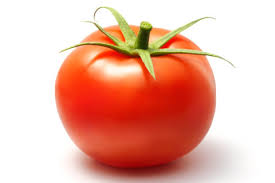# I am a tomato ^_^

Probability Level 5Out of all the permutations of the letters of the word "tomatoes" , the permutations in which 2nd O is the 5th letter of the word, are $\dfrac{a}{b}$ times of total. (The ratio of number of these special permutations to the number of all permutations is $\dfrac{a}{b}$ ) where $\mathrm{gcd(a,b)}= 1$. Find the value of $a+b$

$\bullet$ This problem is a part of the set Vegetable combinatorics

×